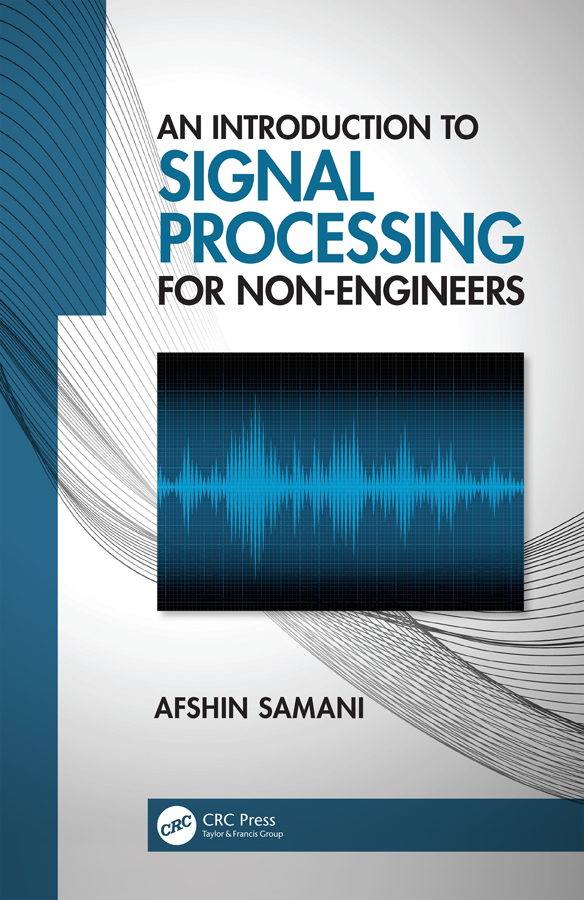# An Introduction to Signal Processing for Non-Engineers

## 1st Edition

CRC Press

128 pages | 49 B/W Illus.

Hardback: 9780367207557
pub: 2019-11-07
SAVE ~\$15.99
Available for pre-order
\$79.95
\$63.96
x

FREE Standard Shipping!

### Description

This book introduces the main and basic concepts of signal processing for scientists and students with no engineering background. The book presents the concepts with minimum use of mathematical formulations and more emphasis on visual illustrations. The idea is to present an intuitive approach to understand the basis of signal processing and exemplify some practical applications of the concepts by which the readers achieve basic knowledge and skills in signal processing. Most of illustrations in the book have been created by computer programming in MATLAB, thus the reader will learn the basis of using computer in signal processing applications.

1. Introduction

1.1 Why do we need to simplify the theories of signal processing?

1.2 What is a signal?

1.3 What is noise?

2. Pipeline of measurement

2.1 Sensors

2.2 Amplification

2.3 Analog to digital conversion

3. Time and frequency representation of continuous time signals

3.1 Periodic signals

3.2 Fourier series

3.3 Fourier transform

3.4 Duality

3.5 Signal energy

4. Sampling of continuous time signals

4.1 Discrete time signals

4.2 Sampling in the frequency domain

4.3 Nyquist criterion

4.4 Practical consideration

5. Discrete Fourier transform

5.1 Frequency resolution

5.2 Fast Fourier transform

5.3 Aliasing in time domain

6. Power spectrum

6.1 Stationarity

6.2 Auto-correlation function

6.3 Spectral estimation

6.3.1 Non-parametric methods

6.3.2 Parametric methods

6.4 Signal characteristics based on the power spectrum

7. Systems and their properties

7.1 System properties

7.2 Linear time invariant systems

7.3 The system response and differential and difference equations

7.4 Transfer function and differential and difference equations

7.5 The system response in the frequency domain

7.6 Stability and the transfer function

7.7 The power spectrum of System input and output

7.8 The transfer function and parametric estimation of power spectrum

8. Filters

8.1 Ideal frequency response of filters

8.2 Filter order

8.3 Filter design

8.4 Filter phase response

8.5 Implementation of filters

8.6 Spatial filters

Appendix A.1: A brief introduction to MATLAB

Appendix A.2: Complex numbers

Appendix A.3: An introduction to convolution

Appendix A.4: Correlation

References

Index